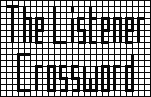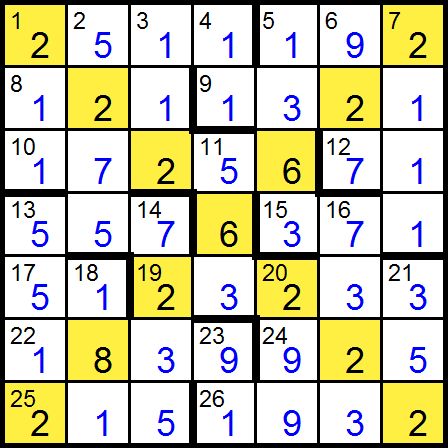## Listener Crossword 4138: Solution NotesBack to 2011 menu## The X-factor by IOA

### Puzzle explanation

The letters correspond to the numbers 1 to 26 in the order AXOKRBUZDYHJVCTQNFGIEMLPSW. The digits in the completed grid, shown here in full, read in natural order, decode as SHADE EVENS BUT RUB OUT ALL OF ODDS: ERGO X.

### Puzzle solution process

The grid numbers shared by across and down entries (where one always has 3 digits while the other has 4) are 1, 5 and 13, which must correspond to A, R and V in some order.

Clue K means H has 2 digits and K is one of {4, 12, 17, 23}. The R clue with HHH thus gives a 4-digit answer, so the other R clue means OKKK has 3 digits, so K = 4.

Clue U’s answer is a bit less than UUUU; the only possible entries with the right number of digits are 7dn = 2111 and 9ac = 5917 (not 5ac = 529, because there’s only one U clue).

Since A and V are both odd, the answer to the second V clue is even, whether X is even or odd. If V is 5, then the answer has a factor of 5 (V), so it ends in 0, which isn’t allowed for either 5ac or 5dn. If V is 1, the answer to L is either 7 or 9 (U), which doesn’t have enough digits for any entry. Thus, V = 13.

From the first V clue (whose answer has 3 or 4 digits), O < 7. It can’t be 1 or 5 (taken by A and R) or 4 (taken by K). If O is 6, the first V clue gives 9059 (if U is 7) or 11649 (if U is 9), neither of which fits at 13ac or 13dn. Clue O is QU, which can have at most 3 digits, so it can’t be 2dn. Thus, O = 3.

From clue W, XXXXX < WW, which can be at most 676, so X = 2 (not 1, because there’s only one X clue, and not 3 because it’s taken by O).

The second R clue now gives 192, which means the first R clue has a 4-digit answer starting with 1, so H is 11 or 12 (not 10, since 4dn can’t end with 0). The first R answer is thus either 1363 or 1760, but neither 1ac nor 5dn can end with 0, so H = 11. This is entered at 4dn, so 9ac starts with 1, which rules out 9ac = 5917 (above), so U = 7 (7dn = 2111). Also, 1ac ends in 1, so it can’t be the first R answer, thus R = 5 (5ac = 192, 5dn = 1363) and A = 1.

The two V clues now give 557 and 5512 for 13ac and 13dn respectively.

The second A clue is (2D + 13)DD, so to avoid having more than 4 digits, D must be at most 15; the available values are {6, 8, 9, 10, 12, 14, 15}. D can’t be 6 because that would make its clue, 16DD + S, give 576 + S for 6dn, where we know the first digit is 9. For the remainder, the answer to clue D has 4 digits, which rules out all but 9 and 14. We know the first digit of 14dn is 7, so it can’t be 3136 + S, so D isn’t 14; thus D = 9, which gives 1ac = 2511 for the second A clue.

The D answer is now 9ac = 1296 + S, which must match 13_1, so S is 15 or 25 (not 5, which is taken by R). The X clue (2dn = 5__5) is the first A clue (1dn = 2__) multiplied by S, so S = 25 and 9ac = 1321. The X clue now gives 25(BB + 21B + 49) = 5__5; if B is 8, we get 7025, which is too high, so B = 6, 2dn = 5275 and 1dn = 211.

Y’s answer is now 17256, which must be either 10ac or 19ac, so 11dn either begins with 5 or ends with 7. Its clue (H) is WW − 113, so if it ends in 7, W must be 20, but that would give 20dn = 1104, which doesn’t fit. So Y = 10 (10ac = 17256). To match 11dn = 5__, W = 26 (not 25, which is taken by S), 11dn = 563 and 26ac = 1932.

The M value is 1839, which now fits only at 22ac, so M = 22. This makes 23dn = 91, which is the L value, so L = 23. The P value is 925, which now fits only at 6dn or 24ac, but 6 is already taken, so P = 24. The F value is 181, which now fits only at 18dn, so F = 18. The T value is 371, which fits only at 15ac, so T = 15, making 6dn = 927. The G value is now 23233, which fits only at 19ac, so G = 19. That makes the C value 7235, which fits only at 14dn, so C = 14.

The only remaining place for the Z value of 121 is 8ac, so Z = 8 and the grid is complete. From the O clue (3dn), 112 = 7Q, so Q = 16. From the Q clue (16dn), 7323 = II + 6923, so I = 20. From the I clue (20dn), 299 = 320 − E, so E = 21. The remaining unassigned entries are 12ac = 71 and 17ac = 51, of which only the latter can be the N value of 3N, so N = 17 and J = 12.

The grid digits, in row order, with the immediately forced letter breaks, are:
25, 1119, 212113, 2117, 25, 6, 7, 15, 5, 7, 6, 3, 7, 15, 123, 23, 3, 18, 3, 9, 9, 25, 215, 19, 3, 2.

Converting the forced digits to the corresponding letters, we get:
25, 1119, 212113, 2117, 25, B, U, 15, R, U, B, O, U, 15, 123, 23, O, 18, O, D, D, 25, 215, 19, O, X. With a bit of trial and error, this leads to SHADE EVENS BUT RUB OUT ALL OF ODDS, ERGO X.

Back to 2011 menu